# How to Make Negative Numbers Red in Excel

In daily work, we often mark positive number in green and negative numbers in red when calculating increasing values and decreasing values. Mark negative numbers in red color can make users lookup negative numbers immediately by one glance, so, it is useful of us to learn how to make negative numbers red in worksheet. Actually, there are some tricks to make negative numbers red in excel, this article will introduce you some of them step by step.

Precondition:

Prepare a table with increase and decrease values. Some of them are negative numbers.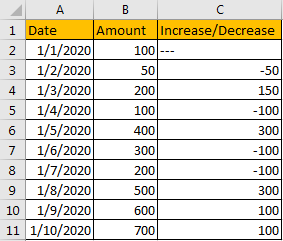Now we want to make all negative numbers in red.

## Method 1: Make All Negative Numbers Red by Format Cells Setting

Step 1: Select the list contains negative numbers, then right click to load menu. Click Format Cells on menu.Step 2: On Format Cells, under Number tab, click Custom, then under Type enter 0;[Red]-0. Then click OK to confirm update.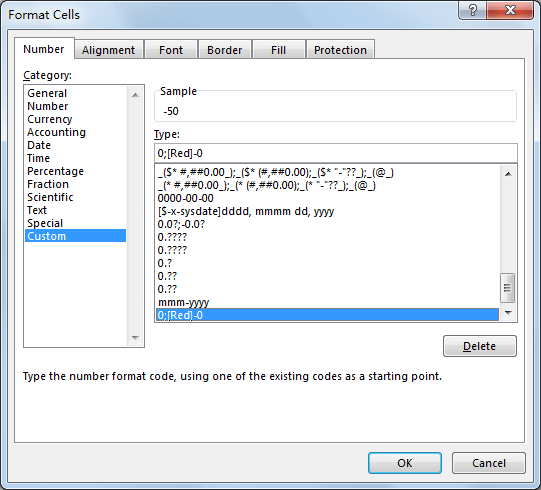Step 3: Verify that after above setting, all negative numbers are marked in red properly.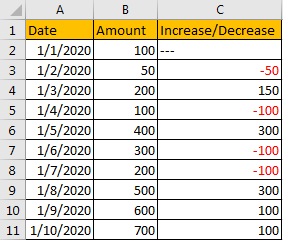Comment:

If the number is displayed in percentage like -2.35%, you can enter 0.00%;[Red]-0.00% under Type. For other number types, you can just add [Red] before its type.

## Method 2: Make All Negative Numbers Red by Conditional Format

Step 1: Select the list contains negative numbers.

Step 2: Click Home in ribbon, click Conditional Formatting in Styles group.Step 3: Select New Rule in Conditional Formatting menu.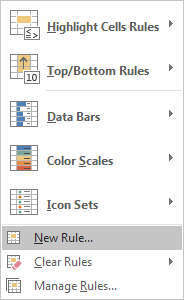Step 4: In New Formatting Rule screen, select ‘Format only cells that contain”, then in Format only cells with section, select ‘Cell Value’, ‘Less Than’ in dropdown lists, and type ‘0’ in last textbox refer to below screenshot. Then click Format button in Preview section.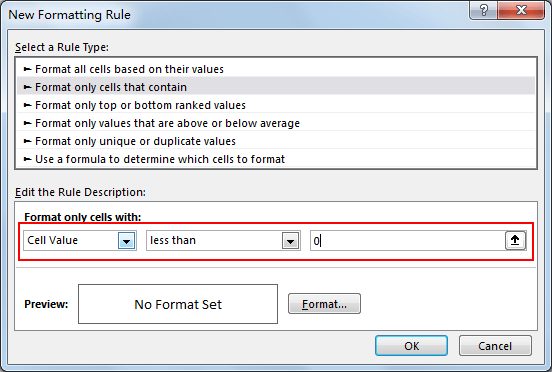Step 5: In Format Cells screen, set negative number format per your demands. In this article, we only want to make them in red color, so in Color dropdown list, select red. Then click OK.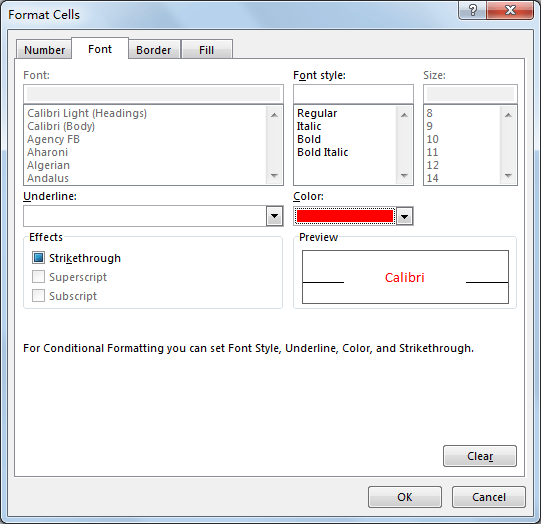Step 6: In New Formatting Rule screen, you can see defined format in Preview. Then click OK.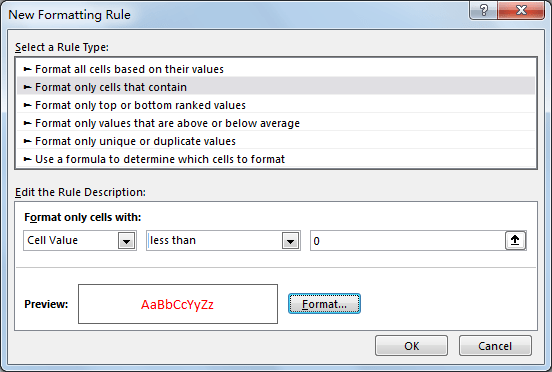Step 7: Then you find that all negative numbers are marked in red properly.Related Posts

Cap percentage values between 0 and 100

This article will talk about how to cap the percentage values between 0% and 100% in Microsoft Excel Spreadsheet or Google Sheets. If you are a newbie on Excel or google Sheets, you may be able to do this by ...

Calculate Cap Percentages to Specific Value

This article will talk about how to limit the cap percentage of a given amount to a given value in Microsoft Excel Spreadsheet or Google Sheets. If you are a newbie on Excel or google Sheets, you may be able ...

Calculate Win Loss Tie

Suppose you got a task to calculate the win, loss, and tie totals; what would you do? If you are new to Ms Excel and don't have enough experience with it, then you might do this task manually but let ...

Calculate Years Between Dates In Ms Excel

If you are an avid Ms Excel user, then you might have come across a task in which you needed to calculate the years between the dates; you might take it easy and do this task manually, which is also ...

Calculate Number of Hours between Two Times

Calculating the difference between two times might be a valuable statistic for subsequent computations or averages, whether you're producing a time sheet for staff or recording personal exercises. While Excel has a plethora of complex functions, including date and time ...

Calculate Loan Interest in Given Year

When you borrow money, you are supposed to repay it gradually. Lenders, on the other hand, want to be compensated for their services and the risk they incur by lending you money. That is, you will not just repay the ...

Calculate Interest Rate for Loan

The interest rate is the fee charged by a lender to a borrower and is expressed as a percentage of the principal—the lent amount. The interest rate on a loan is often expressed as an annual percentage rate, abbreviated as ...

Calculate Interest for Given Period

Using the IPMT function in Excel, we can compute the interest payment on any loan. This step-by-step tutorial will guide Excel users of all skill levels through the process to calculate interest for given period. Finally, the formula: =IPMT(B3/12,1,B5,-B2) The ...

How To Use Excel GCD Function

This post will guide you how to use Excel GCD function with syntax and examples in Microsoft excel. Description The Excel GCD function Returns the greatest common divisor of two or more integers. So you can use the GCD function ...

Calculate A Ratio From Two Numbers In Excel

In elementary mathematics, a ratio is a connection or comparison between two or more integers. For example, ratios are often expressed as ":" to demonstrate the relationship between two numbers. You would think that manually calculating a ratio from two ...

Sidebar